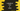# C++ program to find a value in a linkedList## How to find a value in a linkedList :

In this post, we will learn how to find one value in a linkedList in C++. We will write one program that will create one linked list, display the list and find one value in that list based on a user input.

## C++ program :

Below is the complete C++ program :

``````#include <iostream>
using namespace std;

struct Node
{
int value;
Node *next;
};

{

public:
{
Node *node = new Node;
node->value = value;

{
node->next = NULL;
}
else
{
}
}

void displayList()
{
while (flag != NULL)
{
cout << flag->value << endl;
flag = flag->next;
}
}

bool search(int value)
{

while (flag != NULL)
{
if (flag->value == value)
{
return true;
}
flag = flag->next;
}
return false;
}
};

int main()
{
int total, value, searchValue;

cout << "Enter the total nodes :" << endl;
cin >> total;

for (int i = 0; i < total; i++)
{
cout << "Enter value for node " << i << " :" << endl;
cin >> value;
}

cout << "Printing the linked list :" << endl;

cout << "Enter a value to search in this linked list :" << endl;
cin >> searchValue;

{
cout << "Found !!" << endl;
}
else
{
}

return 0;
}``````

### Explanation :

• In this example, we have one class LinkedList to handle all linked list operations.
• displayList method is used to display the linked list.
• search method is used to search an item in a linked list. This method take one integer value as its argument and returns true or false i.e. one boolean value based on the value is found in the linked list or not.
• Based on the result of search, it prints one message.

### Sample Output :

``````Enter the total nodes :
5
Enter value for node 0 :
1
Enter value for node 1 :
3
Enter value for node 2 :
4
Enter value for node 3 :
6
Enter value for node 4 :
7
7
6
4
3
1
Enter a value to search in this linked list :
10

Enter the total nodes :
5
Enter value for node 0 :
1
Enter value for node 1 :
3
Enter value for node 2 :
5
Enter value for node 3 :
7
Enter value for node 4 :
9